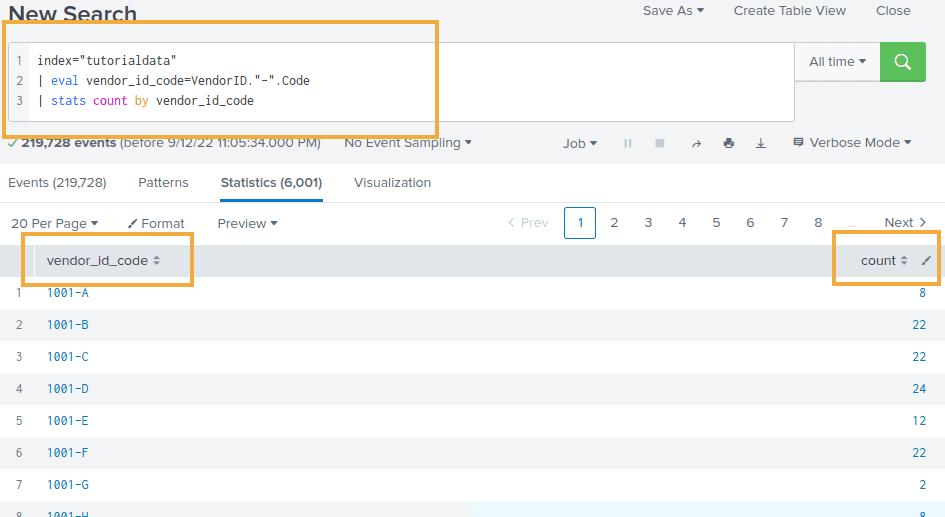Splunk Groupby: Examples with Stats

# Splunk Groupby: Examples with Stats

Last updated:

For info on how to use rex to extract fields: Splunk regular Expressions: Rex Command Examples

Group-by in Splunk is done with the stats command.

General template: search criteria | extract fields if necessary | stats or timechart

## Group by count

Use stats count by field_name

Example: count occurrences of each field my_field in the query output:

source=logs "xxx"
| rex "my\-field: (?<my_field>[a-z]) "
| stats count by my_field
| sort -count


## Group by count, by time bucket

Use timechart count by field_name instead of stats

source=logs "xxx"
| rex "my\-field: (?<my_field>[a-z]) "
| timechart span=30m count by my_field


## Group by averages and percentiles, time buckets

The field must be numeric

You can have multiple aggregations in a single pass:

Use timechart with timechart and aggregation functions such as avg, p50, p90, p99, etc:

source=logs "xxx"
| rex "my\-numeric-\-field: (?<my_numeric_field>[0-9]+) "
| timechart span=30m avg(my_numeric_field), p90(my_numeric_field), p99(my_numeric_field)


## Group by count distinct, time buckets

How many unique values for my_field for each 1-minute bucket?

source=logs "xxx"
| rex "my\-field: (?<my_field>[a-z]) "
| timechart span=1m distinct_count(my_field)


## Group by sum

Use stats sum(value_field_name) by group_field_name

Sample logs

... customer="john doe" order_value=20 ...
... customer="mary white" order_value=25 ...
... customer="john doe" order_value=100 ...


Example query Sum the total order value for each different customer:

source=order_logs
| rex " customer=\"(?<customer>[^\"]+)\" "
| rex " order_value=(?<order_value>[0-9]+) "
| stats sum(order_value) as sum_order_value by customer_id
| sort -sum_order_value


Result:

|---customer----|---sum_order_value--|
| john doe      | 120                |
| mary white    | 25                 |


## Group by multiple fields

All examples use the tutorial data from Splunk running on a local Splunk version

Combine both fields using eval and then use stats:

Example: group by count Vendor ID and Code, together:

index="tutorialdata"
| eval vendor_id_code=VendorID."-".Code
| stats count by vendor_id_codeJust build a new field using eval and . and group on that